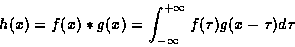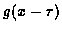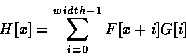Next: 13.3.2.1 Two-Dimensional Convolutions Up: 13.3 Convolutions Previous: 13.3.1 Introduction

### 13.3.2 The Convolution Operation

The convolution operation is a mathematical operation which takes two functions f(x) and g(x) and produces a third function h(x). Mathematically, convolution is defined as:(13)

g(x) is referred to as the filter. The integral only needs to be evaluated over the range whereis nonzero (called the support of the filter).

In spatial domain image processing, you discretize the operation. f(x) becomes an array of pixels F[x]. The kernel g(x) is an array of values G[0...(width-1)] (assume finite support). Equation 13 becomes:(14)

David Blythe
1999-08-06